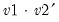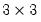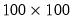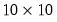Command Reference : Matrix Language Reference

 @outer
Outer product of vectors or series.
Syntax: @outer(v1, v2)
v1: vector, series
v2: vector, series
Return: matrix
Returns the outer product of the two vectors or series. If used with two vectors, @outer requires that v1 and v2 be the same size.
If used with two series, @outer returns a square matrix of every possible product of the elements of the two inputs.
If used with two vectors, @outer computes. If v1 and v2 are column vectors, the result is the square matrix of every possible product of the elements of the two inputs. If v1 and v2 are row vectors, the result is a scalar containing the sum of the element products.
Examples
vector v1 = @fill(1, 2, 3)
vector v2 = @fill(4, 5, 6)
matrix m1 = @outer(v1, v2)
produces amatrix of outer products M1,
workfile u 100
series x = nrnd
series y = nrnd
matrix m2 = @outer(x, y)
creates an undated workfile with 100 observations, amatrix of outer products M1,
If you wish to compute the outer product of series for a subset of observations, you will first have to extract the data to a sub-vector:
smpl 1 10
stom(x, vx)
stom(y, vy)
matrix m3 = @outer(vx, vy)
matrix m4 = m2.@sub(@range(1, 10), @range(1, 10))
produces identicalouter products for the first 10 observations in the workfile in M3 and M4.
Cross-references